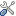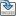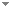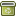# The dependence of computability on numerical notations

Synthese 198 (11):10485-10511 (2020)

 Abstract Which function is computed by a Turing machine will depend on how the symbols it manipulates are interpreted. Further, by invoking bizarre systems of notation it is easy to define Turing machines that compute textbook examples of uncomputable functions, such as the solution to the decision problem for first-order logic. Thus, the distinction between computable and uncomputable functions depends on the system of notation used. This raises the question: which systems of notation are the relevant ones for determining whether a function is computable? These are the acceptable notations. I argue for a use criterion of acceptability: the acceptable notations for a domain of objects D\documentclass[12pt]{minimal} \usepackage{amsmath} \usepackage{wasysym} \usepackage{amsfonts} \usepackage{amssymb} \usepackage{amsbsy} \usepackage{mathrsfs} \usepackage{upgreek} \setlength{\oddsidemargin}{-69pt} \begin{document}$${\mathcal {D}}$$\end{document} are the notations that we can use for the usual D\documentclass[12pt]{minimal} \usepackage{amsmath} \usepackage{wasysym} \usepackage{amsfonts} \usepackage{amssymb} \usepackage{amsbsy} \usepackage{mathrsfs} \usepackage{upgreek} \setlength{\oddsidemargin}{-69pt} \begin{document}$${\mathcal {D}}$$\end{document}-activities. Acceptable notations for natural numbers are ones that we can count with. Acceptable notations for logical formulas are the ones that we can use in inference and logical analysis. And so on. This criterion makes computability a relative notion—whether a function is computable depends on which notations are acceptable, which is relative to our interests and abilities. I argue that this is not a problem, however, since there is independent reason for taking the extension of computable function to be relative to contingent facts. Similarly, the fact that the use criterion makes a mathematical distinction depend on practical concerns is also not a problem because it dovetails with similar phenomena in other areas of computability theory, namely the roles of notation in computation over real numbers and of Extended Church’s Thesis in complexity theory. Keywords No keywords specified (fix it) Categories No categories specified (categorize this paper) ISBN(s) DOI 10.1007/s11229-020-02732-x OptionsEdit this recordMark as duplicateExport citationFind it on ScholarRequest removal from indexRevision history

PhilArchive copy

Upload a copy of this paper     Check publisher's policy     Papers currently archived: 72,577

Setup an account with your affiliations in order to access resources via your University's proxy server
Configure custom proxy (use this if your affiliation does not provide a proxy)

## References found in this work BETA

Introduction to Metamathematics.Stephen Cole Kleene - 1952 - Princeton, NJ, USA: North Holland.
On Computable Numbers, with an Application to the Entscheidungsproblem.Alan Turing - 1936 - Proceedings of the London Mathematical Society 42 (1):230-265.
Computability & Unsolvability.Martin Davis - 1958 - Dover Publications.
Visual Thinking in Mathematics: An Epistemological Study.Marcus Giaquinto - 2007 - Oxford, England: Oxford University Press.

## Similar books and articles

The Role of Notations in Mathematics.Carlo Cellucci - 2020 - Philosophia 48 (4):1397-1412.
Church's Thesis and the Conceptual Analysis of Computability.Michael Rescorla - 2007 - Notre Dame Journal of Formal Logic 48 (2):253-280.
The Representational Foundations of Computation.Michael Rescorla - 2015 - Philosophia Mathematica 23 (3):338-366.
Lp -Computability.Ning Zhong & Bing-Yu Zhang - 1999 - Mathematical Logic Quarterly 45 (4):449-456.
Decoding Gentzen's Notation.Luca Bellotti - 2018 - History and Philosophy of Logic 39 (3):270-288.
Church's Thesis Without Tears.Fred Richman - 1983 - Journal of Symbolic Logic 48 (3):797-803.
Computability and Recursion.Robert I. Soare - 1996 - Bulletin of Symbolic Logic 2 (3):284-321.

## Analytics

Added to PP index
2020-06-18

Total views
11 ( #858,866 of 2,533,592 )